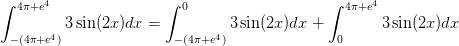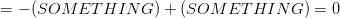# AP Calculus AB : Use of the Fundamental Theorem to represent a particular antiderivative, and the analytical and graphical analysis of functions so defined

## Example Questions

### Example Question #1 : Integrals

Evaluate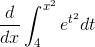.

Does not exist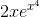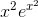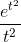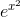Explanation:

Even though an antideritvative of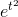does not exist, we can still use the Fundamental Theorem of Calculus to "cancel out" the integral sign in this expression.. Start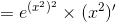. You can "cancel out" the integral sign with the derivative by making sure the lower bound of the integral is a constant, the upper bound is a differentiable function of,, and then substituting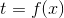in the integrand. Lastly the Theorem states you must multiply your result by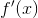(similar to the directions in using the chain rule).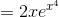.

### Example Question #2 : Integrals

The graph of a function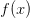is drawn below. Select the best answers to the following: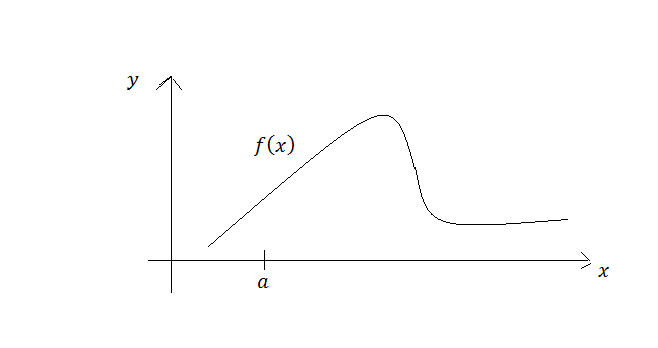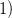What is the best interpretation of the function?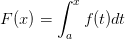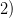Which plot shows the derivative of the function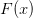?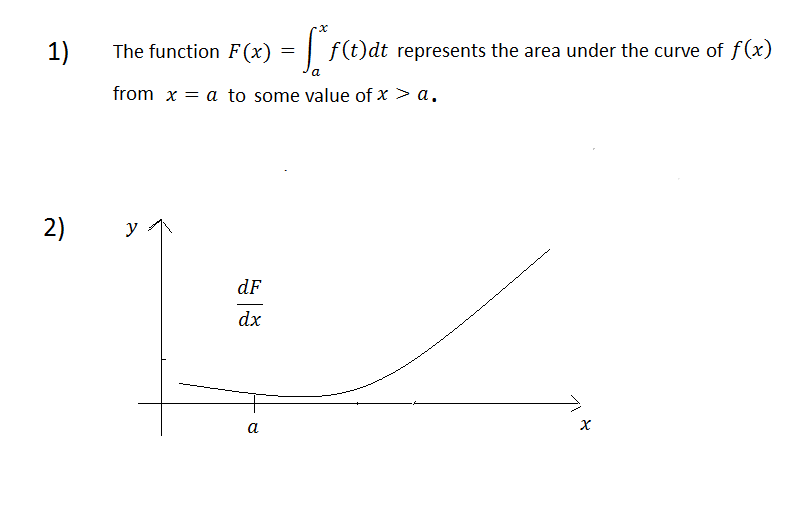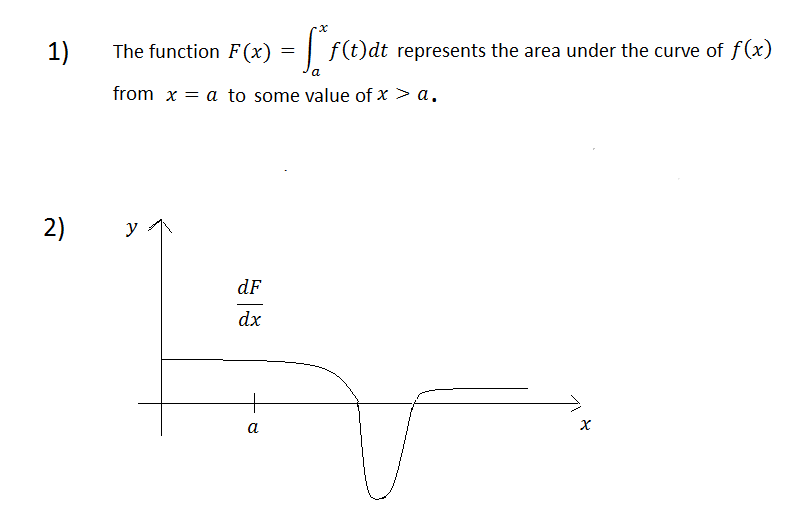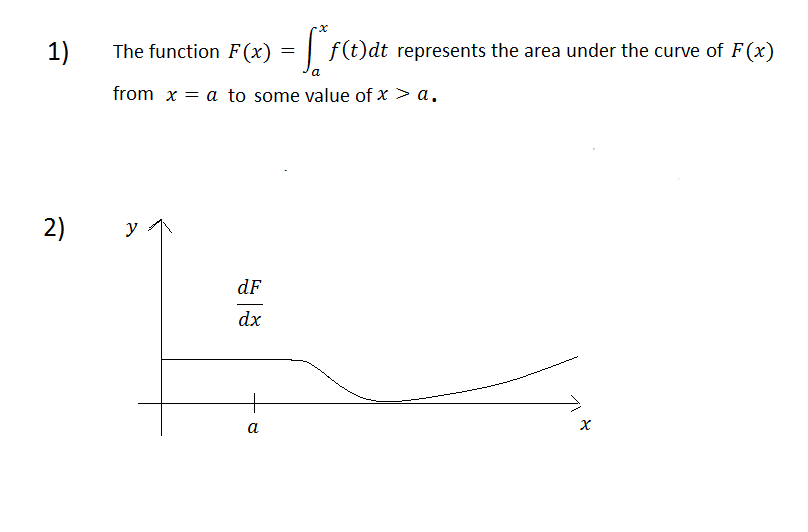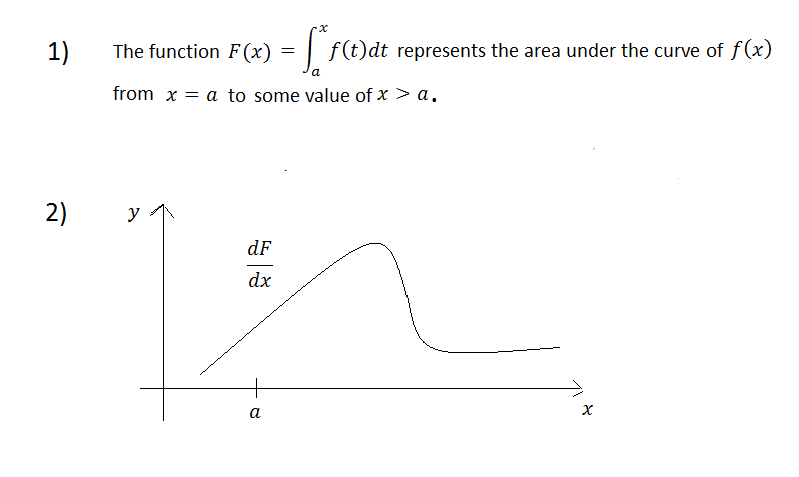Explanation:The functionrepresents the area under the curvefromto some value of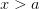.

Do not be confused by the use ofin the integrand. The reason we useis because are writing the area as a function of, which requires that we treat the upper limit of integration as a variable. So we replace the independent variable ofwith a dummy indexwhen we write down the integral. It does not change the fundamental behavior of the functionor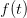The graph of the derivative ofis the same as the graph for. This follows directly from the Second Fundamental Theorem of Calculus.

If the functionis continuous on an interval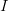containing, then the function defined by:has for its' derivative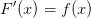### Example Question #1 : Integrals

Evaluate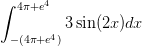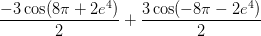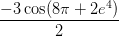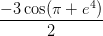Explanation:

Here we could use the Fundamental Theorem of Calculus to evaluate the definite integral; however, that might be difficult and messy.

Instead, we make a clever observation of the graph of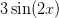Namely, that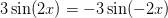This means that the values of the graph when comparing x and -x are equal but opposite. Then we can conclude that